Case Based Questions Test: Circles

# Case Based Questions Test: Circles

Test Description

## 15 Questions MCQ Test Mathematics (Maths) Class 10 | Case Based Questions Test: Circles

Case Based Questions Test: Circles for Class 10 2023 is part of Mathematics (Maths) Class 10 preparation. The Case Based Questions Test: Circles questions and answers have been prepared according to the Class 10 exam syllabus.The Case Based Questions Test: Circles MCQs are made for Class 10 2023 Exam. Find important definitions, questions, notes, meanings, examples, exercises, MCQs and online tests for Case Based Questions Test: Circles below.
Solutions of Case Based Questions Test: Circles questions in English are available as part of our Mathematics (Maths) Class 10 for Class 10 & Case Based Questions Test: Circles solutions in Hindi for Mathematics (Maths) Class 10 course. Download more important topics, notes, lectures and mock test series for Class 10 Exam by signing up for free. Attempt Case Based Questions Test: Circles | 15 questions in 30 minutes | Mock test for Class 10 preparation | Free important questions MCQ to study Mathematics (Maths) Class 10 for Class 10 Exam | Download free PDF with solutions
 1 Crore+ students have signed up on EduRev. Have you?
Case Based Questions Test: Circles - Question 1

### Read the following text and answer the following question on the basis of the same:A farmer has a field in the shape of triangle with AB = 13 cm, BC = 14 cm and AE = 7 cm. He wants to leave a space in the form of a circular field for growing wheat and the remaining for growing vegetables.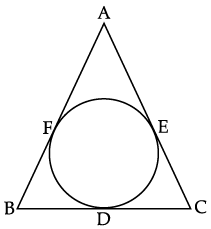Q. The measure of BF is:

Detailed Solution for Case Based Questions Test: Circles - Question 1

∴ AB = 13 cm (given)

∴ BF = AB – AF

= (13 – 7) cm

AE = 7 cm (given)

Then, AF = AE (Tangents of the circle)

⇒ AF = 7 cm.

[Proved, AF = 7 cm]

BF = 6 cm

Case Based Questions Test: Circles - Question 2

### Read the following text and answer the following question on the basis of the same:A farmer has a field in the shape of triangle with AB = 13 cm, BC = 14 cm and AE = 7 cm. He wants to leave a space in the form of a circular field for growing wheat and the remaining for growing vegetables.Q. The measure of CE + CD is:

Detailed Solution for Case Based Questions Test: Circles - Question 2

∴ AB = 13 cm (given)

∴ BF = AB – AF

BC = 14 cm (given)

= (13 – 7) cm

AE = 7 cm (given)

Then, AF = AE (Tangents of the circle)

⇒ AF = 7 cm.

[Proved, AF = 7 cm]

BF = 6 cm

BF = 6 cm (Proved)

∴ BD = BF (Tangents of the circle)

So, BD = 6 cm.

and BD = 6 cm [Proved]

then CD = BC – BD

= (14 – 6) cm = 8 cm

but CD = CE (Tangents of the circle)

∴ CE = 8 cm

So, CD + CE = (8 + 8) cm = 16 cm.

Case Based Questions Test: Circles - Question 3

### Read the following text and answer the following question on the basis of the same:A farmer has a field in the shape of triangle with AB = 13 cm, BC = 14 cm and AE = 7 cm. He wants to leave a space in the form of a circular field for growing wheat and the remaining for growing vegetables.Q. The measure of AF is:

Detailed Solution for Case Based Questions Test: Circles - Question 3 AE = 7 cm (given)

Then, AF = AE (Tangents of the circle)

⇒ AF = 7 cm.

Case Based Questions Test: Circles - Question 4

Read the following text and answer the following question on the basis of the same:

A farmer has a field in the shape of triangle with AB = 13 cm, BC = 14 cm and AE = 7 cm. He wants to leave a space in the form of a circular field for growing wheat and the remaining for growing vegetables.Q. The measure of BD is:

Detailed Solution for Case Based Questions Test: Circles - Question 4

∴ AB = 13 cm (given)

BF = AB – AF

= (13 – 7) cm

[Proved in Q. 1., AF = 7 cm]

BF = 6 cm

BF = 6 cm (Proved)

∴ BD = BF (Tangents of the circle)

So, BD = 6 cm.

Case Based Questions Test: Circles - Question 5

Read the following text and answer the following question on the basis of the same:

A farmer has a field in the shape of triangle with AB = 13 cm, BC = 14 cm and AE = 7 cm. He wants to leave a space in the form of a circular field for growing wheat and the remaining for growing vegetables.Q. The measure of AC is:

Detailed Solution for Case Based Questions Test: Circles - Question 5

∴ AB = 13 cm (given)

BF = AB – AF

= (13 – 7) cm

AE = 7 cm (given)

Then, AF = AE (Tangents of the circle)

⇒ AF = 7 cm.

[Proved AF = 7 cm]

BF = 6 cm

BC = 14 cm (given)

and BD = 6 cm [Proved in Q. 3]

then CD = BC – BD

= (14 – 6) cm = 8 cm

but CD = CE (Tangents of the circle)

∴ CE = 8 cm

So, CD + CE = (8 + 8) cm = 16 cm.

CE = 8 cm

and AE = 7 cm (given)

then, AC = AE + EC

= (7 + 8) cm

= 15 cm.

Case Based Questions Test: Circles - Question 6

Both Assertion and Reason are true but Reason is not correct explanation of Assertion

In the following figure, a circle with centre O is inscribed in a quadrilateral ABCD such that, it touches the sides BC, AB, AD and CD at points P, Q, R and S respectively. If AB = 29 cm, AD = 23 cm, ∠B = 90° and DS = 5 cm.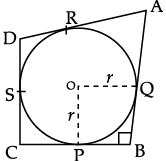Q. Find the value of AR:

Detailed Solution for Case Based Questions Test: Circles - Question 6

Here, AD = 23 cm (given)

and

Here, DS = 5 cm

but DR = DS (Length of tangents are equal)

then DR = 5 cm.

DR = 5 cm (Proved)

then AR = AD – DR cm

= (23 – 5) cm = 18 cm.

Case Based Questions Test: Circles - Question 7

Both Assertion and Reason are true but Reason is not correct explanation of Assertion

In the following figure, a circle with centre O is inscribed in a quadrilateral ABCD such that, it touches the sides BC, AB, AD and CD at points P, Q, R and S respectively. If AB = 29 cm, AD = 23 cm, ∠B = 90° and DS = 5 cm.Q. Find the value of BP:

Detailed Solution for Case Based Questions Test: Circles - Question 7

AB = 29 cm (given) but AQ = AR(Length of tangents are equal) then AQ = 18 cm

Here, AD = 23 cm (given)

and

Here, DS = 5 cm

but DR = DS (Length of tangents are equal)

then DR = 5 cm.

DR = 5 cm (Proved)

then AR = AD – DR cm

= (23 – 5) cm = 18 cm. [Proved]

∴ BQ = AB – AQ

= (29 – 18) cm = 11 cm.

BQ = 11 cm [Proved]

and BP = BQ (Length of tangents are equal)

∴ BP = 11 cm.

Case Based Questions Test: Circles - Question 8

Both Assertion and Reason are true but Reason is not correct explanation of Assertion

In the following figure, a circle with centre O is inscribed in a quadrilateral ABCD such that, it touches the sides BC, AB, AD and CD at points P, Q, R and S respectively. If AB = 29 cm, AD = 23 cm, ∠B = 90° and DS = 5 cm.Q. Find the value of DR:

Detailed Solution for Case Based Questions Test: Circles - Question 8 Here, DS = 5 cm

but DR = DS

(Length of tangents are equal)

then DR = 5 cm.

Case Based Questions Test: Circles - Question 9

Both Assertion and Reason are true but Reason is not correct explanation of Assertion

In the following figure, a circle with centre O is inscribed in a quadrilateral ABCD such that, it touches the sides BC, AB, AD and CD at points P, Q, R and S respectively. If AB = 29 cm, AD = 23 cm, ∠B = 90° and DS = 5 cm.Q. Find the value of QB:

Detailed Solution for Case Based Questions Test: Circles - Question 9

AB = 29 cm (given)

but AQ = AR (Length of tangents are equal)

then AQ = 18 cm

Here, AD = 23 cm (given)

and

Here, DS = 5 cm

but DR = DS (Length of tangents are equal)

then DR = 5 cm.

DR = 5 cm (Proved)

then AR = AD – DR cm

= (23 – 5) cm = 18 cm. [Proved]

∴ BQ = AB – AQ

= (29 – 18) cm = 11 cm.

Case Based Questions Test: Circles - Question 10

Both Assertion and Reason are true but Reason is not correct explanation of Assertion

In the following figure, a circle with centre O is inscribed in a quadrilateral ABCD such that, it touches the sides BC, AB, AD and CD at points P, Q, R and S respectively. If AB = 29 cm, AD = 23 cm, ∠B = 90° and DS = 5 cm.Q. AR is equal to:

Detailed Solution for Case Based Questions Test: Circles - Question 10 AR = AQ (Length of tangents are equal)
Case Based Questions Test: Circles - Question 11

Read the following text and answer the following questions on the basis of same: A ferris wheel (Or a big wheel in the United Kingdom) is an amusement ride consisting of a rotating upright wheel with multiple passenger carrying components attached to the rim in such a way that as the wheel turns, they are kept upright, usually by gravity.

After taking a ride in Ferris wheel Aarti came out from the crowd and was observing her friends who were enjoying the ride. She was curios about the different angles and measures that wheel will form. She forms the figure as given below: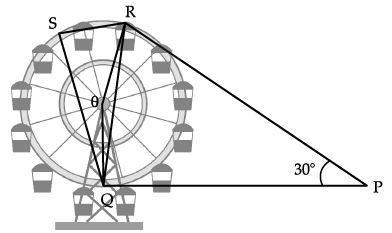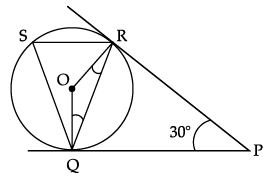Q. Find ∠RQP

Detailed Solution for Case Based Questions Test: Circles - Question 11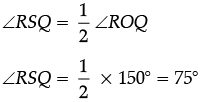∠RSQ =∠RQP = 75°

[Angles in alternate segment]

Case Based Questions Test: Circles - Question 12

Read the following text and answer the following questions on the basis of same: A ferris wheel (Or a big wheel in the United Kingdom) is an amusement ride consisting of a rotating upright wheel with multiple passenger carrying components attached to the rim in such a way that as the wheel turns, they are kept upright, usually by gravity.

After taking a ride in Ferris wheel Aarti came out from the crowd and was observing her friends who were enjoying the ride. She was curios about the different angles and measures that wheel will form. She forms the figure as given below:Q. Find ∠ORP

Detailed Solution for Case Based Questions Test: Circles - Question 12 Radius is always perpendicular to the tangent.
Case Based Questions Test: Circles - Question 13

Read the following text and answer the following questions on the basis of same: A ferris wheel (Or a big wheel in the United Kingdom) is an amusement ride consisting of a rotating upright wheel with multiple passenger carrying components attached to the rim in such a way that as the wheel turns, they are kept upright, usually by gravity.

After taking a ride in Ferris wheel Aarti came out from the crowd and was observing her friends who were enjoying the ride. She was curios about the different angles and measures that wheel will form. She forms the figure as given below:Q. In the given figure, Find ∠ROQ

Detailed Solution for Case Based Questions Test: Circles - Question 13 ∠ROQ +∠RPQ = 180°

∠ROQ + 30° = 180°

∠ROQ = 150°

Case Based Questions Test: Circles - Question 14

Read the following text and answer the following questions on the basis of same: A ferris wheel (Or a big wheel in the United Kingdom) is an amusement ride consisting of a rotating upright wheel with multiple passenger carrying components attached to the rim in such a way that as the wheel turns, they are kept upright, usually by gravity.

After taking a ride in Ferris wheel Aarti came out from the crowd and was observing her friends who were enjoying the ride. She was curios about the different angles and measures that wheel will form. She forms the figure as given below:Q. Find ∠RSQ

Detailed Solution for Case Based Questions Test: Circles - Question 14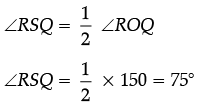Case Based Questions Test: Circles - Question 15

Read the following text and answer the following questions on the basis of same: A ferris wheel (Or a big wheel in the United Kingdom) is an amusement ride consisting of a rotating upright wheel with multiple passenger carrying components attached to the rim in such a way that as the wheel turns, they are kept upright, usually by gravity.

After taking a ride in Ferris wheel Aarti came out from the crowd and was observing her friends who were enjoying the ride. She was curios about the different angles and measures that wheel will form. She forms the figure as given below:Q. Find ∠ORQ

Detailed Solution for Case Based Questions Test: Circles - Question 15 In triangle ORQ,

∠ORQ + ∠OQR + ∠ROQ= 180°

2∠ORQ = 180° – ∠ROQ

2∠ORQ = 180° – 150° = 30°

∠ORQ = 15°

## Mathematics (Maths) Class 10

53 videos|406 docs|138 tests
 Use Code STAYHOME200 and get INR 200 additional OFF Use Coupon Code
Information about Case Based Questions Test: Circles Page
In this test you can find the Exam questions for Case Based Questions Test: Circles solved & explained in the simplest way possible. Besides giving Questions and answers for Case Based Questions Test: Circles, EduRev gives you an ample number of Online tests for practice

## Mathematics (Maths) Class 10

53 videos|406 docs|138 tests